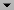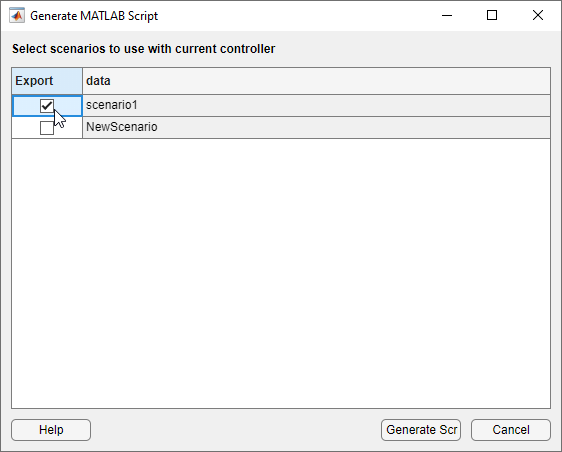## Generate MATLAB Code from MPC Designer

This topic shows how to generate MATLAB® code for creating and simulating model predictive controllers designed in the MPC Designer app. Generated MATLAB scripts are useful when you want to programmatically reproduce designs that you obtained interactively.

To create a MATLAB script:

1. In the MPC Designer app, interactively design and tune your model predictive controller.

2. On the Tuning tab, in the Analysis section, click the Export Controller arrow.

Alternatively, on the MPC Designer tab, in the Result, click Export Controller.

Note

If you opened MPC Designer from Simulink®, click the Update and Simulate arrow.

3. Under Export Controller or Update and Simulate, click `Generate Script`.

4. In the Generate MATLAB Script dialog box, select one or more simulation scenarios to include in the generated script.5. Click to create the MATLAB script for creating the current MPC controller and running the selected simulation scenarios. The generated script opens in the MATLAB Editor.

In addition to generating a script, the app exports the following to the MATLAB workspace:

• A copy of the plant used to create the controller, that is the controller internal plant model

• Copies of the plants used in any simulation scenarios that do not use the default internal plant model

• The reference and disturbance signals specified for each simulation scenario